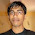### Cube in a sphere

Source: CMU Spring 2010 Course on Great Theoretical Ideas in Computer Science

Problem: 10% of the surface of a sphere is colored green, and the rest is colored blue. Show that no matter how the colors are arranged, it is possible to inscribe a cube in the sphere so that all of its vertices are blue.

Hint: Use probabilistic analysis. Consider a random cube and calculate the expected number of vertices that are blue.

(Update 23/06/10):
Solution: Posted by connect2ppl - Giridhar Addepalli (CSE, IITK alumnus and Yahoo! Sr. Software Engineer) in comments!

1.Nice! Reminded me of the various problems that I solved on the 'Probabilistic Method' recently.

2.Didn't solve it, just wondering if 12.5% is the threshold percentage for this to happen.

12.5 = 100/#vertices

3.@sangharsh.. Yes.. its correct..

@infiniti (Nishant Totla) .. It would be good if you could share some of those problems here :)

4.for a random cube, expected number of blue vertices is (9/10)*8 = 7.2

how to go from here?

if probability of all vertices being blue is +ve, then it implies the existence of a cube we r looking for.

But, how to get red of dependency among the colors of vertices??

5.oops!!

expectation being 7.2 itself proves the existence of cubes where all vertices are blue ( since max value of random variable is 8, 7.2 expected value is possible only bcoz of the existence of cases where its value is 8)

6.@Giridhar.. Thanks.. Correct solution :)

7.shouldn't the expected number of blue vertexes be
8*[(0.9)^8] + 7*[(0.9)^7][(0.1)^1] +......+1*[(0.9)^1][(0.1)^7] rather than 8*(0.9) ?
The first one sums up to around 4.